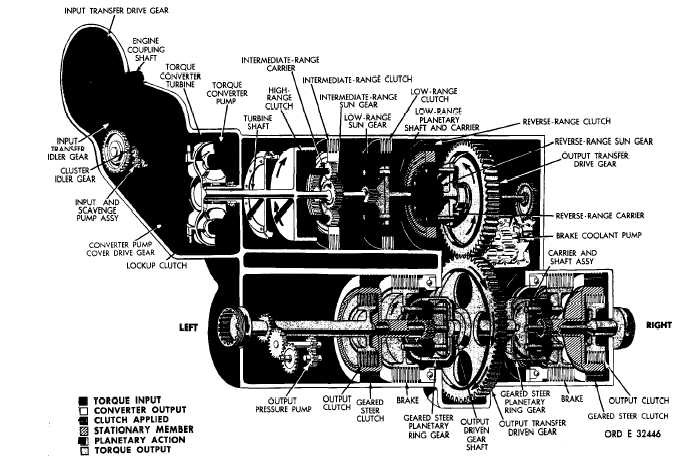Custom SearchC H A P    2,   SEC  V T O R Q U E     P A T H S PAR  5  1 51.   TORQUE   PATH   –   REVERSE   1   GEAR, CONVERTER   OPERATION   (fig.   27) .   T o r q u e    i s    t r a n s m i t t e d    t o    t h e    t u r b i ne shaft   in   the   manner   described   in   pars.   42   and 43,   above.   In   reverse   range,   torque   is   trans- mitted    from    the    turbine    shaft    to    the    output t r a n s f e r    d r i v e    g e a r    b y    a    c o m p o u n d    a r r a n g e- m e n t     o f     t h e     r e v e r s e - r a n g e     a n d     l o w - r a n ge p l a n e t a r y    g e a r s. .   The   input   member   of   this   compound   ar- rangement   is   the   low-range   sun   gear.   Torque is   transmitted   to   the   low-range   pinions   which rotate   in   the   direction   opposite   the   sun   gear. T h e    s l o w e r    m o v i n g ,    l o w - r a n g e    c a r r i e r ,    a l- though    it    is    rotating,    is    a    reaction    member. T h e    l o w - r a n g e    r i n g    g e a r ,    m e s h e d    w i t h    i ts pinions,   rotates   in   the   direction   opposite   the sun   gear.   Thus,   the   reversal   of   rotation   ac- tually   occurs   in   the   low-range   planetary. Figure   27.   Reverse   1-gear   torque   path,   converter   operation .   T h e     r e v e r s e - r a n g e     s u n     g e a r     i s     a t- tached   to   the   low-range   ring   gear   and   rotates with   it.   The   reverse-range   clutch   is   engaged, holding   the   reverse-range   ring   gear   stationary. T h e    r e v e r s e - r a n g e    p l a n e t a r y    p i n i o n s    a r e    in mesh   with   both   the   ring   gear   and   sun   gear. T h e    r o t a t i o n    o f    t h e    r e v e r s e - r a n g e    s u n    g e ar forces   the   pinions   to   rotate   within   the   station- ary   ring   gear.   This   drives   the   reverse-range c a r r i e r     i n     t h e     s a m e     d i r e c t i o n     a s ,     b u t     a t     a slower   speed   than,   the   sun   gear.   The   reverse- range    carrier,    splined    to    the    low-range    plane- tary   carrier   shaft,   drives   the   output   transfer d r i v e    g e a r. .   T h e    r e m a i n d e r    o f    t h e    t o r q u e    p a t h    is i d e n t i c a l    t o    t h a t    d e s c r i b e d    f o r    f i r s t    g e a r    in par.   47b   and   c,   a b o v e .    R e f e r    t o    T A B U L A T ED D A T A ,    p a r .    7 ,    f o r    g e a r    r a t i o s    a p p l i c a b l e    to r e v e r s e    1    g e a r. 5 4Integrated Publishing, Inc. - A (SDVOSB) Service Disabled Veteran Owned Small Business